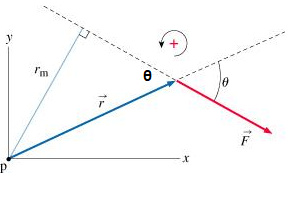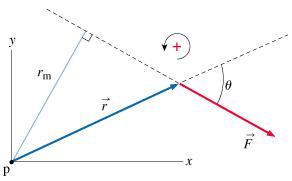# Problem: What is the length, rm, of the moment arm of the force F about point p in terms of r and θ?

###### FREE Expert Solution

From trigonometry:Using angle properties, the vertically opposite angle is equal to θ.

81% (353 ratings)###### Problem DetailsWhat is the length, rm, of the moment arm of the force F about point p in terms of r and θ?